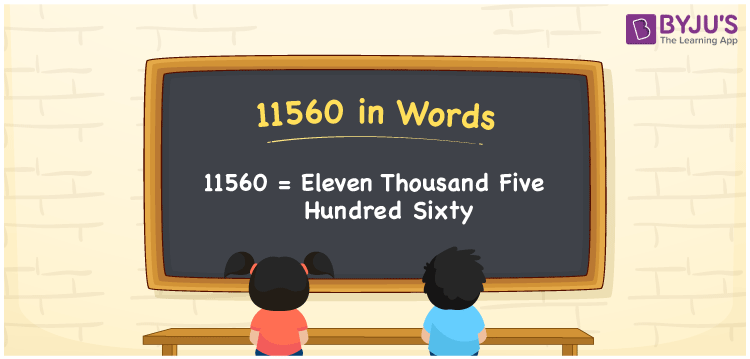# 11560 in Words

The number name of 11560 is “Eleven thousand five hundred sixty”. For converting numbers into words, we generally employ the place value system. Also, the number 11560 is a natural and a cardinal number. For example, Rahul purchased clothes worth Rs. 11560. It can also be written as “Rahul purchased the clothes worth Rs. Eleven thousand five hundred sixty”.

 11560 in Words: Eleven Thousand Five Hundred Sixty. Eleven Thousand Five Hundred Sixty in Numerical Form: 11560.

## 11560 in English Words## How to Write 11560 in Words?

To write the number 11560 in words, use the below place value table.

 Ten-thousands Thousands Hundreds Tens Ones 1 1 5 6 0

The expanded form of 11560 is as follows:

= 1 × Ten thousand + 1 × Thousand + 5 × Hundred + 6 × Ten + 0 × One

= 1 × 10000 + 1 × 1000 + 5 × 100 + 6 × 10 + 0 × 1

= 10000 + 1000 + 500 + 60

= 11560

= Eleven thousand five hundred sixty

Hence, 11560 in words is eleven thousand five hundred sixty.

11560 in words – Eleven thousand five hundred sixty

Is 11560 an odd number? – No

Is 11560 an even number? – Yes

Is 11560 a perfect square number? – No

Is 11560 a perfect cube number? – No

Is 11560 a prime number? – No

Is 11560 a composite number? – Yes

## Frequently Asked Questions on 11560 in Words

Q1

### How to spell 11560 in words?

11560 in words is eleven thousand five hundred sixty.

Q2

### Simplify 11000 + 560, and express it in words.

Simplifying 11000 + 560, we get 11560. Hence, 11560 in words is eleven thousand five hundred sixty.

Q3

### Is 11560 an odd number?

No, 11560 is not an odd number.